# Gibbs phenomenon

A characteristic of the behaviour of the partial sums (or their averages) of a Fourier series.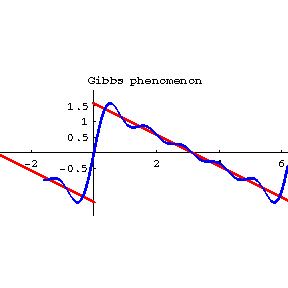Figure: g044410a

First noted by H. Wilbraham  and rediscovered by J.W. Gibbs  at a much later date. Let the partial sumsof the Fourier series of a functionconverge toin some neighbourhood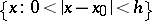of a pointat which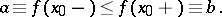The Gibbs phenomenon takes place foratifwhere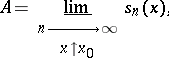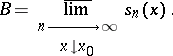The geometrical meaning of this is that the graphs (cf. Fig.) of the partial sumsdo not approach the "expected" intervalon the vertical line, but approach the strictly-larger intervalasand. The Gibbs phenomenon is defined in an analogous manner for averages of the partial sums of a Fourier series when the latter is summed by some given method.

For instance, the following theorems are valid for-periodic functionsof bounded variation on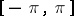.

1) At points of non-removable discontinuity, and only at such points, the Gibbs phenomenon occurs for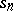. In particular, if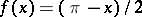for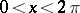, then for the point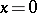the segment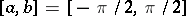, while the segment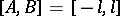where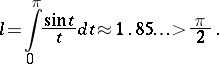2) There exists an absolute constant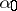,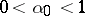, such that the Cesàro averages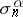do not have the Gibbs phenomenon if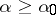, while if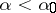the phenomenon is observed at all points of non-removable discontinuity of.